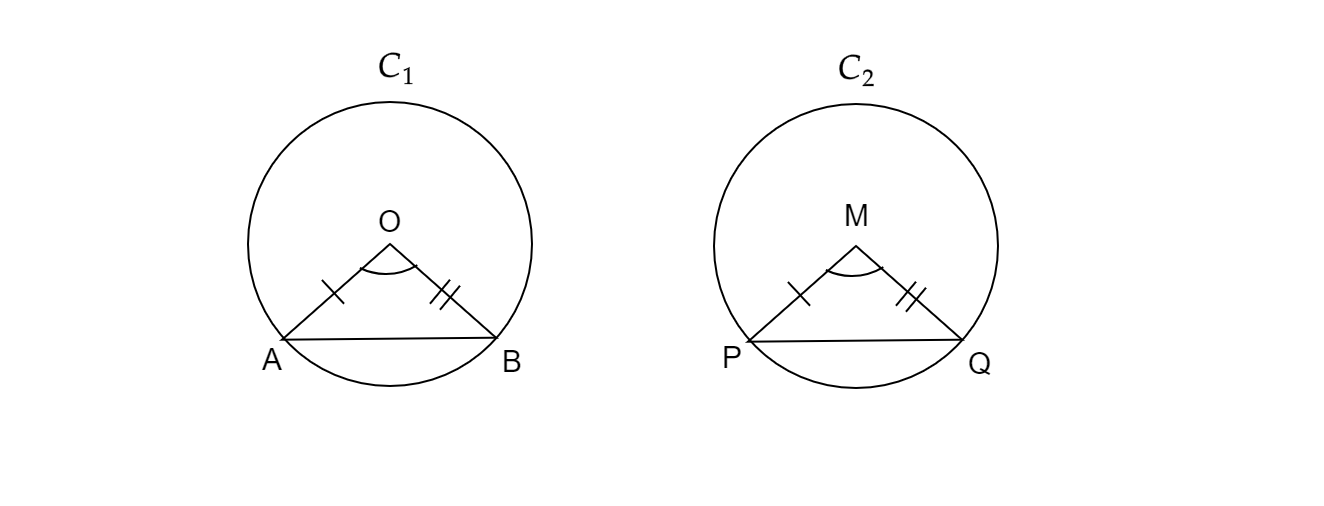# Prove that if chords of congruent circles subtend equal angles at their centres, then the chords are equal.

Given:

Chords of congruent circles subtend equal angles at their centres.

To do:

We have to prove that the chords are equal.

Solution:Let $c_{1}$ and $C_{2}$ be two congruent circles, $AB$ and $PQ$ are their chords respectively.

Let us join $OA$ and $OB$ in circle $C_{1}$.

Similarly, in cirlcle $C_{2}$, join $MP$ and $MQ$.

In $\vartriangle OAB$ and $\vartriangle MPQ$.

$OA=MP$                                [$\because$ Radius of the congruent circles are same]

$OB=MQ$                               [$\because$ Radius of congruent circles are same]

$\angle AOB=\angle PMQ$              [It is given chords of congruent circles subtend equal angles at their centres]

$\Rightarrow \vartriangle OAB\cong \vartriangle MPQ$                [SAS rule of congruency]

$\therefore AB=PQ$                           [By CPCT rule]

Hence, it has been proved that the chords are equal.

Updated on: 10-Oct-2022

27 Views